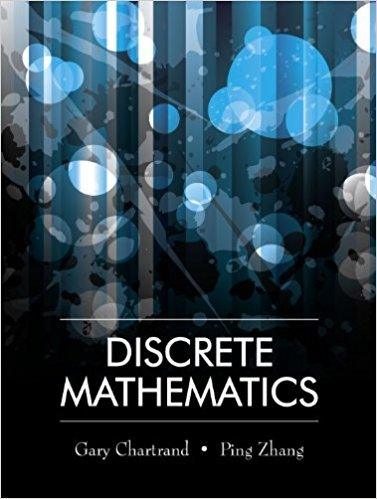×
×

# Solutions for Chapter 3: Methods of Proof## Full solutions for Discrete Mathematics | 1st Edition

ISBN: 9781577667308Solutions for Chapter 3: Methods of Proof

Solutions for Chapter 3
4 5 0 358 Reviews
29
0
##### ISBN: 9781577667308

This expansive textbook survival guide covers the following chapters and their solutions. This textbook survival guide was created for the textbook: Discrete Mathematics, edition: 1. Discrete Mathematics was written by and is associated to the ISBN: 9781577667308. Since 39 problems in chapter 3: Methods of Proof have been answered, more than 12861 students have viewed full step-by-step solutions from this chapter. Chapter 3: Methods of Proof includes 39 full step-by-step solutions.

Key Math Terms and definitions covered in this textbook
• Adjacency matrix of a graph.

Square matrix with aij = 1 when there is an edge from node i to node j; otherwise aij = O. A = AT when edges go both ways (undirected). Adjacency matrix of a graph. Square matrix with aij = 1 when there is an edge from node i to node j; otherwise aij = O. A = AT when edges go both ways (undirected).

• Cofactor Cij.

Remove row i and column j; multiply the determinant by (-I)i + j •

• Column space C (A) =

space of all combinations of the columns of A.

• Companion matrix.

Put CI, ... ,Cn in row n and put n - 1 ones just above the main diagonal. Then det(A - AI) = ±(CI + c2A + C3A 2 + .•. + cnA n-l - An).

• Diagonal matrix D.

dij = 0 if i #- j. Block-diagonal: zero outside square blocks Du.

• Distributive Law

A(B + C) = AB + AC. Add then multiply, or mUltiply then add.

• Indefinite matrix.

A symmetric matrix with eigenvalues of both signs (+ and - ).

• Independent vectors VI, .. " vk.

No combination cl VI + ... + qVk = zero vector unless all ci = O. If the v's are the columns of A, the only solution to Ax = 0 is x = o.

• Kirchhoff's Laws.

Current Law: net current (in minus out) is zero at each node. Voltage Law: Potential differences (voltage drops) add to zero around any closed loop.

• Krylov subspace Kj(A, b).

The subspace spanned by b, Ab, ... , Aj-Ib. Numerical methods approximate A -I b by x j with residual b - Ax j in this subspace. A good basis for K j requires only multiplication by A at each step.

• Least squares solution X.

The vector x that minimizes the error lie 112 solves AT Ax = ATb. Then e = b - Ax is orthogonal to all columns of A.

• Linear transformation T.

Each vector V in the input space transforms to T (v) in the output space, and linearity requires T(cv + dw) = c T(v) + d T(w). Examples: Matrix multiplication A v, differentiation and integration in function space.

• Norm

IIA II. The ".e 2 norm" of A is the maximum ratio II Ax II/l1x II = O"max· Then II Ax II < IIAllllxll and IIABII < IIAIIIIBII and IIA + BII < IIAII + IIBII. Frobenius norm IIAII} = L La~. The.e 1 and.e oo norms are largest column and row sums of laij I.

• Plane (or hyperplane) in Rn.

Vectors x with aT x = O. Plane is perpendicular to a =1= O.

• Rank one matrix A = uvT f=. O.

Column and row spaces = lines cu and cv.

• Semidefinite matrix A.

(Positive) semidefinite: all x T Ax > 0, all A > 0; A = any RT R.

• Symmetric factorizations A = LDLT and A = QAQT.

Signs in A = signs in D.

• Transpose matrix AT.

Entries AL = Ajj. AT is n by In, AT A is square, symmetric, positive semidefinite. The transposes of AB and A-I are BT AT and (AT)-I.

• Tridiagonal matrix T: tij = 0 if Ii - j I > 1.

T- 1 has rank 1 above and below diagonal.

• Vector space V.

Set of vectors such that all combinations cv + d w remain within V. Eight required rules are given in Section 3.1 for scalars c, d and vectors v, w.

×